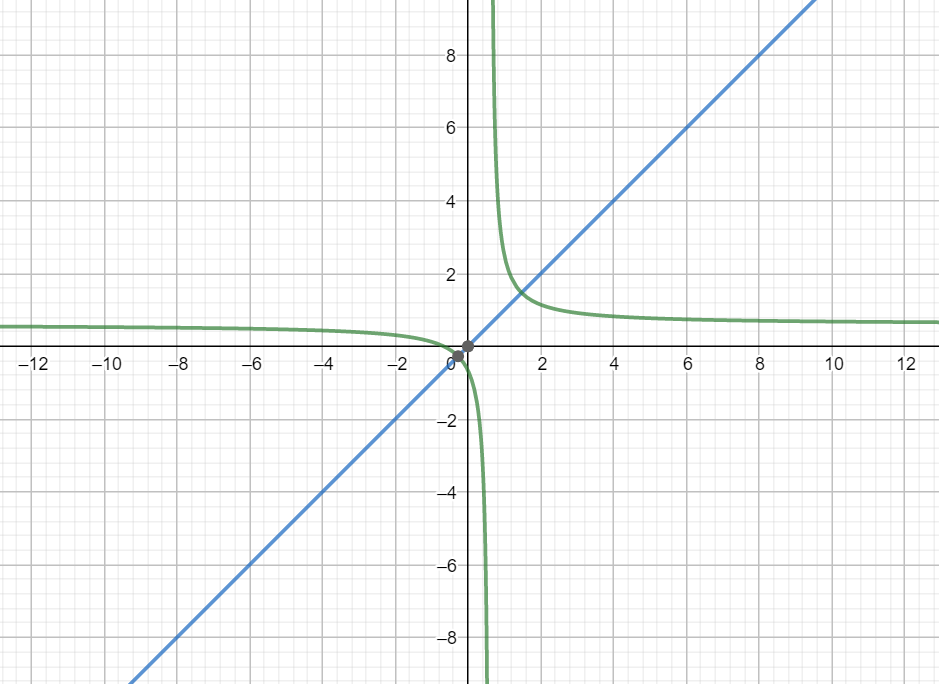Courses
Courses for Kids
Free study material
Free LIVE classes
MoreLIVE
Join Vedantu’s FREE Mastercalss

# Let $f:R-\left\{ \dfrac{3}{5} \right\}\to R$ be defined by $f(x)=\dfrac{3x+2}{5x-3}$ . Then,which of the following options are correct?.A. ${{f}^{-1}}(x)=f(x)$B. ${{f}^{-1}}(x)=-f(x)$C. $fof(x)=-x$D. ${{f}^{-1}}(x)=\dfrac{1}{19}f(x)$Verified
361.5k+ views
Hint: To find an inverse function such as f(x) we have the following method: Express x in terms of f(x) and then replace x with g(x) and f(x) with x. The resultant function g(x) will be the inverse of the function f(x) then we check which of the options are correct.

We have the function $f(x)=\dfrac{3x+2}{5x-3}$ . First of all let us express x in terms of f(x). For that we have,
$f(x)[5x-3]=3x+2$
Multiplying f(x) we have,
$5xf(x)-3f(x)=3x+2$
Taking 3x in LHS and -3f(x) in RHS we have,
$5xf(x)-3x=3f(x)+2$
Taking x common from the terms in LHS we have,
$x(5f(x)-3)=3f(x)+2$
Dividing both sides with coefficient of x we have,
$x=\dfrac{3f(x)+2}{5f(x)-3}$
Now replacing x with g(x) and f(x) with x we have,
$g(x)=\dfrac{3x+2}{5x-3}$
This function g(x) is the inverse of the function f(x). Hence, we can write ${{f}^{-1}}(x)=\dfrac{3x+2}{5x-3}$ .
We had $f(x)=\dfrac{3x+2}{5x-3}$ and ${{f}^{-1}}(x)=\dfrac{3x+2}{5x-3}$ . Therefore $f(x)={{f}^{-1}}(x)$ .
Hence, option A is the correct answer.
Note: We should know that the inverse of a function is a mirror image of the function about the line $y=x$ means if we were to plot the graph of a function and its inverse we will find that they are mirror image of each other about the line $y=x$ .This the graph of the function $f(x)=\dfrac{3x+2}{5x-3}$ . As we can see the function is perfectly symmetric and if we were to draw the mirror image it would again give the same function.
Last updated date: 02nd Oct 2023
Total views: 361.5k
Views today: 7.61k